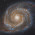## Saturday, June 23, 2012

### Python: Calculating the average color of an area of an image (PIL)

Here's a snippet of code I whipped up in Python to calculate the the average color of a square shaped area of an image.  I used the Python Imaging Library (PIL) to load the image, so be sure to have it available if you're using this.

```import Image

def get_average_color((x,y), n, image):
""" Returns a 3-tuple containing the RGB value of the average color of the
given square bounded area of length = n whose origin (top left corner)
is (x, y) in the given image"""

r, g, b = 0, 0, 0
count = 0
for s in range(x, x+n+1):
for t in range(y, y+n+1):
pixlr, pixlg, pixlb = image[s, t]
r += pixlr
g += pixlg
b += pixlb
count += 1
return ((r/count), (g/count), (b/count))

r, g, b = get_average_color((24,290), 50, image)
print r,g,b

```
This is great for detecting the color of an area of an animated and constantly changing game screen, where finding the color of a single pixel may not be accurate enough for your needs.

1.Hey, thanks for the snippet!

Just a small error though. You reference the 'pix' variable on line 8 which you haven't created yet. I'm assuming you mean to either pass in 'pix' instead of 'image' or create 'pix' by doing 'pix = image.load()' within your function.

Cheers!

1.Good catch!

Updated the snippet to use the correct argument and added some example usage below for context. Thanks!

2.You can also use scipy and get average in just one line:

from scipy import misc
3.